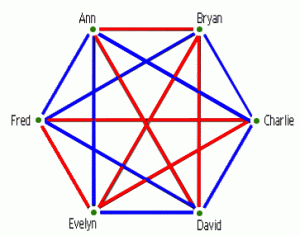# Party At Ramsey’s“Why mathematics?”  is a question that greets me on occasion from friends and acquaintances wanting to delve into a casual conversation about the subject.   Many times I will start out by offering a recreational math example, such as the Six Person Party Problem from Ramsey Graph Theory.    This is a great problem to present, because, unlike other maths that deal with jargon such as Meromorphic Functions , Hermitian Operators ,(or insert your favorite years-to-understand abstract math term here), Ramsey Graph Theory, and a lot of related Graph Theory in general, can be approached in the beginning with a grasp of high school algebra and a little mathematical maturity.

Basically,  imagine yourself at a party with five other people.  At this party  there is either some three that are mutual friends or some three that are mutual strangers.   This is always the case with a group of six or more people.     One can easily prove this  in an informal conversation style by modeling the party with a graph.

A graph in Graph Theory is not the same as a graph of some function f(x) that is usually seen in a course on calculus.  Rather, it is a set of “points” (just as  you would think of a dot on a piece of paper) that we call vertices or nodes and a set of edges (think straight line segments) such that every edge has two nodes, one at each end, and there is a reflexive relation between the nodes and edges.   This is a general definition of a classic graph.  The nodes and edges can abstractly represent a great variety of relations.

A complete graph is a graph such that every two nodes is connected via one edge.  Thus, a complete graph on six nodes (the nodes representing the six people) can be used to model our party.  Now, if we pick one node, call it A,  it is connected to the other five with five distinct edges by the given definition of a complete graph.  Furthermore, if I take two crayons, red  for friendship and blue for strangers, and started coloring at random each edge one of the two colors, then I will always get some three of the five that share the same color.  This is an example of a powerful tool in combinatorics known as the Pigeonhole Principle.

Pick the three edges that have the same color and let them all be red.  Since the graph is a complete graph, the end nodes B, C, and D, of the three edges, are connected to A and to each other as well.  Therefore, any two of B,C, and D with A form a triangle and B, C, and D forms a triangle by themselves also. Triangles in Graph Theory are the same as a complete graph with three nodes.

If I start coloring the edges of the triangle formed by B, C, and D randomly red and blue, then an edge will be colored red, in which case there will be a  red monochromatic triangle formed by A and the two end nodes of B,C, and D that share the common red edge.  However, if we tried to avoid this and color all the edges of the triangle formed by B,C, and D blue, then we have a blue monochromatic triangle formed by the nodes B, C, and D.  A red monochromatic triangle represents three mutual friends and a blue monochromatic triangle represents three mutual strangers.  Therefore, as you can see we have proved, using a graph as a model, that at a party of six or more people there always exists some three people who are mutual friends or mutual strangers.

This property of always having a forced monochromatic triangle of one color or the other when coloring the edges randomly with two colors (known as 2-coloring) is not true for a complete graph with five nodes.  Therefore, the minimum number of nodes needed to force a monochromatic triangle of one color or the other is six and is represented by the Ramsey notation R(3,3)=6, where R(k,k) is known as a Diagonal Ramsey Number.   Using this notation,  mathematicians know that R(4,4)=18.  But no one knows what R(5,5) or R(6,6) equals.   It is not even feasible to computationally verify them.    Do you know what they equal?  A quick Google search will give you bounds for them and a plethora of technical results.    It is problems like this that I believe help answer the opening question and bring us closer to understanding the beauty of mathematics.